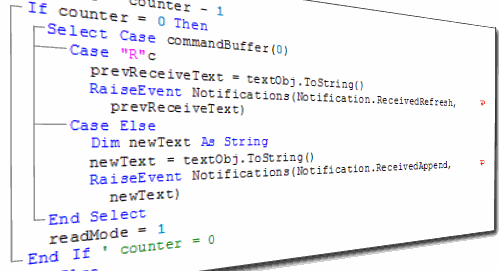# VBA Select Case Statement – Explained

In our previous posts we have talked about Excel If Statement and Excel Nested If’s, and in today’s post we will discuss about VBA select case statement. VBA Select Case can be used instead of complex Excel Nested If statements. This makes the VBA code faster to execute and easier to understand.

Select-Case statement (also called as Switch Case in some languages) checks a variable or an expression for different cases (values). If anyone of the case becomes true then only that case is executed and the program ignores all other cases.

If you remember in our last post we talked about, “how you can change the program flow with VBA MsgBox and case statements”.### Syntax of VBA Select Case Statement:

The Syntax is as under:

`Select Case ConditionCase value_1Code to Execute When Condition = value_1Case value_2Code to Execute When Condition = value_2Case value_3Code to Execute When Condition = value_3Case ElseCode to Execute When all the other cases are FalseEnd Select`

Here, ‘Condition’ refers to the variable or the expression that is to be tested and based on which anyone of the code segments will be executed.

value_1’, ‘value_2’ and ‘value_3’ are the possible outcomes of the ‘Condition’. Whenever anyone of these values matches the ‘Condition’ then its corresponding Case block will execute.

‘Else’ is a kind of default case value, which will only execute when all the above Case statements result into False. ‘Else’ case is optional but generally it is considered a good practice to use it.

### Examples of Select-Case in VBA:

Now let’s move on to some practical examples of case Statements.

Example 1: Select Case Statement with an Expression.

In the below example, we have supplied a condition (i.e. a=b) to the Select Case statement. If this is True then ‘Case True’ block will be executed and if it is False then ‘Case False’ block will execute.

`Sub Select_Case_Example()'Enter the value for variablesa = InputBox("Enter the value for A:")b = InputBox("Enter the value for B:")' Evaluating the expressionSelect Case a = bCase True MsgBox "The expression is TRUE"Case False MsgBox "The expressions is FALSE"End SelectEnd Sub`

Note: In this code InputBox function is used for getting values from user.

Example 2: Case statement to check Text Strings

In this example we will compare text strings in the Case statements. If a match is found then the corresponding case block will execute otherwise the ‘Case Else’ block will execute.

`Sub Select_Case_Example()'Enter the value for variablesfruit_name = InputBox("Enter the fruit name:")' Evaluating the expressionSelect Case fruit_nameCase "Apple" MsgBox "You entered Apple"Case "Mango" MsgBox "You entered Mango"Case "Orange" MsgBox "You entered Orange"Case Else MsgBox "I didn't knew this fruit!"End SelectEnd Sub`

Example 3: Case statement to check numbers

In the below example we will check if the number entered by user is less than or greater than 5.

`Sub Select_Case_Example()'Enter the value for variablesNum = InputBox("Enter any Number between 1 to 10:")' Evaluating the expressionSelect Case NumCase Is < 5 MsgBox "Your Number is less than 5"Case Is = 5 MsgBox "Your Number is Equal to 5"Case Is > 5 MsgBox "Your Number is greater than 5"End SelectEnd Sub`

Note: You can use IS keyword with Case Statement to compare values.

Example 4: Select Case statement to check multiple conditions inside a single case.

In this example we will ask the user to enter any number from 1-10. And then we will check if the number is even or odd by using multiple conditions in the case statement. Notice here I have used a “,” (comma) to compare multiple conditions in a single case.

`Sub Select_Case_Example()'Enter the value for variablesNum = InputBox("Enter any Number between 1 to 10:")' Evaluating the expressionSelect Case NumCase 2, 4, 6, 8, 10 MsgBox "Your Number is Even."Case 1, 3, 5, 7, 9 MsgBox "Your Number is Odd."Case Else MsgBox "Your Number is out of the range."End SelectEnd Sub`

Note: I know that there are easier methods to check if a number is even or odd, but I have used this example only for explaining how you can check multiple conditions inside a single case statement.

Example 5: Case statement to check a continuous range as condition.

Here we will test a continuous range as a condition. We will ask the user to enter any number between 1-10, if the number is between 1 to 5 (including both 1 and 5) then ‘Case 1 To 5’ will be ‘True’, if the number entered by the user is between 6 and 10 (including both 6 and 10) then ‘Case 6 To 10’ will be ‘True’, if both the previous cases are ‘False’ then ‘Case Else’ will be executed.

`Sub Select_Case_Example()'Enter the value for variablesNum = InputBox("Enter any Number between 1 to 10:")' Evaluating the expressionSelect Case NumCase 1 To 5 MsgBox "Your Number between 1 to 5"Case 6 To 10 MsgBox "Your Number between 6 to 10"Case Else MsgBox "Your Number is out of the range."End SelectEnd Sub`About Ankit Kaul